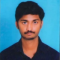## Solve the following equations and verify your answer:(i) $\frac{7x-2}{5x-1}=\frac{7x+3}{5x+4}$(ii) $(\frac{x+1}{x+2})^2=\frac{x+2}{x+4}$Akhileshwar Nani

Updated on 13-Apr-2023 23:45:48

Given:The given equations are:(i) $\frac{7x-2}{5x-1}=\frac{7x+3}{5x+4}$(ii) $(\frac{x+1}{x+2})^2=\frac{x+2}{x+4}$To do:We have to solve the given equations and verify the answers.Solution:To verify the answer we have to find the values of the variables and substitute them in the equation. Find the value of LHS and the value of RHS and check whether both are ... Read More

## Solve the following equations and verify your answer:(i) $\frac{2}{3x}-\frac{3}{2x}=\frac{1}{12}$(ii) $\frac{3x+5}{4x+2}=\frac{3x+4}{4x+7}$Akhileshwar Nani

Updated on 13-Apr-2023 23:24:36

Given:The given equations are:(i) $\frac{2}{3x}-\frac{3}{2x}=\frac{1}{12}$(ii) $\frac{3x+5}{4x+2}=\frac{3x+4}{4x+7}$To do:We have to solve the given equations and verify the answers.Solution:To verify the answer we have to find the values of the variables and substitute them in the equation. Find the value of LHS and the value of RHS and check whether both are ... Read More

## Solve the following equations and verify your answer:(i) $\frac{y-(7-8y)}{9y-(3+4y)}=\frac{2}{3}$(ii) $\frac{6}{2x-(3-4x)}=\frac{2}{3}$Akhileshwar Nani

Updated on 13-Apr-2023 23:23:54

Given:The given equations are:(i) $\frac{y-(7-8y)}{9y-(3+4y)}=\frac{2}{3}$(ii) $\frac{6}{2x-(3-4x)}=\frac{2}{3}$To do:We have to solve the given equations and verify the answers.Solution:To verify the answer we have to find the values of the variables and substitute them in the equation. Find the value of LHS and the value of RHS and check whether both are ... Read More

## Solve the following equations and verify your answer:(i) $\frac{1-9y}{19-3y}=\frac{5}{8}$(ii) $\frac{2x}{3x+1}=1$Akhileshwar Nani

Updated on 13-Apr-2023 23:23:17

Given:The given equations are:(i) $\frac{1-9y}{19-3y}=\frac{5}{8}$(ii) $\frac{2x}{3x+1}=1$To do:We have to solve the given equations and verify the answers.Solution:To verify the answer we have to find the values of the variables and substitute them in the equation. Find the value of LHS and the value of RHS and check whether both are ... Read More

## Solve the following equations and verify your answer:(i) $\frac{2y+5}{y+4}=1$(ii) $\frac{2x+1}{3x-2}=\frac{5}{9}$Akhileshwar Nani

Updated on 13-Apr-2023 23:22:39

Given:The given equations are:(i) $\frac{2y+5}{y+4}=1$(ii) $\frac{2x+1}{3x-2}=\frac{5}{9}$To do:We have to solve the given equations and verify the answers.Solution:To verify the answer we have to find the values of the variables and substitute them in the equation. Find the value of LHS and the value of RHS and check whether both are ... Read More

## Solve the following equations and verify your answer:(i) $\frac{5x-7}{3x}=2$(ii) $\frac{3x+5}{2x+7}=4$Akhileshwar Nani

Updated on 13-Apr-2023 23:21:21

Given:The given equations are:(i) $\frac{5x-7}{3x}=2$(ii) $\frac{3x+5}{2x+7}=4$To do:We have to solve the given equations and verify the answers.Solution:To verify the answer we have to find the values of the variables and substitute them in the equation. Find the value of LHS and the value of RHS and check whether both are ... Read More

## Solve the following equations and verify your answer:(i) $\frac{2x-3}{3x+2}=\frac{-2}{3}$(ii) $\frac{2-y}{y+7}=\frac{3}{5}$Akhileshwar Nani

Updated on 13-Apr-2023 23:20:50

Given:The given equations are:(i) $\frac{2x-3}{3x+2}=\frac{-2}{3}$(ii) $\frac{2-y}{y+7}=\frac{3}{5}$To do:We have to solve the given equations and verify the answers.Solution:To verify the answer we have to find the values of the variables and substitute them in the equation. Find the value of LHS and the value of RHS and check whether both are ... Read More

## Solve the following equation and also check your result: $[(2x+3)+(x+5)]^2+[(2x+3)-(x+5)]^2=10x^2 + 92$Akhileshwar Nani

Updated on 13-Apr-2023 23:20:07

Given:The given equation is $[(2x+3)+(x+5)]^2+[(2x+3)-(x+5)]^2=10x^2 + 92$To do:We have to solve the given equation and check the result.Solution:To check the result we have to find the values of the variables and substitute them in the equation. Find the value of LHS and the value of RHS and check whether both are ... Read More

## Solve each of the following equations and also check your results in each case:(i) $6.5x+(\frac{19.5x-32.5}{2})=6.5x+13+\frac{13x-26}{2}$(ii) $(3x-8)(3x+2)-(4x-11)(2x+1)=(x-3)(x+7)$Akhileshwar Nani

Updated on 13-Apr-2023 23:18:51

Given:The given equations are:(i) $6.5x+(\frac{19.5x-32.5}{2})=6.5x+13+\frac{13x-26}{2}$(ii) $(3x-8)(3x+2)-(4x-11)(2x+1)=(x-3)(x+7)$To do:We have to solve the given equations and check the results.Solution:To check the results we have to find the values of the variables and substitute them in the equation. Find the value of LHS and the value of RHS and check whether both are ... Read More

## Solve each of the following equations and also check your results in each case:(i) $\frac{7x-1}{4}-\frac{1}{3}(2x-\frac{1-x}{2})=\frac{10}{3}$(ii) $0.5\frac{(x-0.4)}{0.35}-0.6(\frac{x-2.71}{0.42})=x+6.1$Akhileshwar Nani

Updated on 13-Apr-2023 23:17:26

Given:The given equations are:(i) $\frac{7x-1}{4}-\frac{1}{3}(2x-\frac{1-x}{2})=\frac{10}{3}$(ii) $0.5\frac{(x-0.4)}{0.35}-0.6(\frac{x-2.71}{0.42})=x+6.1$To do:We have to solve the given equations and check the results.Solution:To check the results we have to find the values of the variables and substitute them in the equation. Find the value of LHS and the value of RHS and check whether both are ... Read More

1 2 3 4 5 ... 21 Next